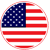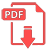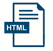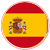# Home | Mathematics | Number Systems

## Convert from Roman to Arabic

• First, specify where you want to convert from. Choose whether you want to convert from Roman to Arabic or from Arabic to Roman.

• Then enter the amount (number) you want to convert. If you convert from Roman, then Roman, if from Arabic, then, of course, Arabic.

• Finally, click the button named Convert. The result of the conversion will immediately appear.

2. How to convert a Roman number to Arabic?

Roman numerals are converted using the following symbols:

M = 1000

D = 500

C = 100

• L = 50

• X = 10

• V = 5

• I = 1

By combining the above Roman numerals you can convert a specific Roman numeral to Arabic. This system uses subtractive notation which means that you can get some of the numbers by using the subtract method. For example, if we want to describe the Arabic numeral four, we can describe it as an Arabic numeral by subtraction as Roman numeral 1 (I) before the Roman numeral (V) as follows: IV. The same method can be used for larger values. For example, the CM stands for 900.

It is important to know that by using the above numbers and using the symbol system described above the largest number that can be represented in this notation is 3,999. With all of this in mind, the converter can handle and convert Roman numerals from 1 to 3999.EnglishSpanish